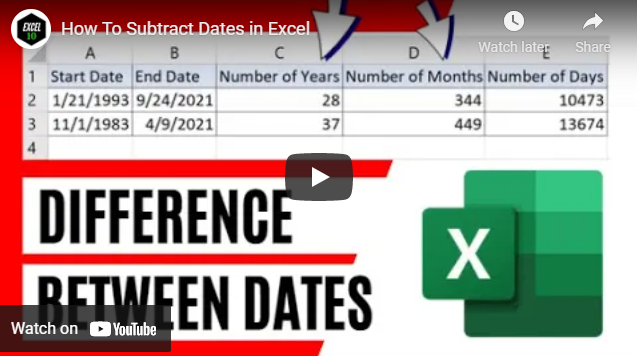# How To Subtract Dates in ExcelIn this Excel tutorial for beginners, you’ll learn how to get the number of days, months, or years between two dates in Microsoft Excel using a formula.

How would you count someone’s age? Simple, by calculating the difference between two dates. There are certain situations where you need to get the number of years, number of months, number of days. There are different ways to get it but the best way to calculate difference between two dates in excel is to use the Dated If function. But the funny thing is that this is a hidden function in excel. You won’t find this function in the formula tab. Even if you write the function excel won’t show the parameter suggestions like it does for other functions. So, you need to understand and use the function on your own. Well, not exactly. I’ll be guiding you. So, lets learn the Function.

The Dated If Formula Looks like this:

= DatedIF(Start_Date, End_Date, "Unit")

Here is the first parameter we have the Starting Date. Write the date or select the date reference here. This date can be in text or date format.

The Second parameter we have the End Date. Write the date or select the date reference here. This date can be in text or date format. But this date can not be smaller than the starting date.

The third parameter is the unit. In the Dated If function there are 6 Units but one of them doesn’t work perfectly. This is why this function is hidden. The units and their explanations are as follows:

"Y" = Subtract dates in excel to get years. For example: IF starting date is 1/21/1993 and End date is 9/24/2021 then the "Y" unit will display 28. That’s 28 years between starting and ending date.

"M" = subtract dates in excel to get months. For example: IF starting date is 1/21/1993 and End date is 9/24/2021 then the "M" unit will display 344. That’s 344 months between starting and ending date.

"D" = subtract dates to get days. For example: IF starting date is 1/21/1993 and End date is 9/24/2021 then the "D" unit will display 10,473. That’s 10,473 days between starting and ending date.

"MD" = This is the unit that doesn’t work properly. It was supposed to calculate difference between days of start and end date, ignoring the months and years. But somehow it doesn’t work properly. For example: IF starting date is 1/21/1993 and End date is 9/24/2021 then the "MD" unit Should display 3. It should calculate difference only in days except months and years. Here the starting date was 21 and the ending date was 24 so the difference is 3.

"YM" = This will calculate the difference between starting date’s month and end date’s month ignoring the days and years. For example: IF starting date is 1/21/1993 and End date is 9/24/2021 then the "YM" unit will display 8. It will calculate difference only in months except days and years. Here the starting date’s month was 1 and the ending date’s month was 9 so the difference is 8.

"YD" = This will calculate the difference between starting date’s days and end date’s days ignoring the Months and years. For example: IF starting date is 1/21/1993 and End date is 9/24/2021 then the "YD" unit will display 246. Because the difference between 21’st January to 24’th September is 246 days. Hope you got the point.

There are a few things you should consider if you are using the DatedIf Function to calculate difference between dates in excel.

1. Your starting date can’t be greater than the ending date, otherwise the formula will display a NUM error

2. Your date format (Both starting date and end date) must be matched will the date format of the computer you are using. Otherwise, you’ll get a Value Error.

3. If you enter date in the formula as text, you must write it within double quotation

Also check this article from Microsoft to learn the known issues of DatedIF function.

This is how I how to subtract two dates to find out difference between two dates in excel. Thanks a million for reading this far. If you can, please support us through Patreon